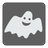Ghosts Age
Elementary+
English HU RU ZH-CN

Nicola takes a moment to study the ghosts. He is trying to think up a new method to determine just how old these ghosts are. He knows that their age is somehow related to their opacity. To measure their opacity Nikola uses a scale of 10000 units to 0 units, where 10000 is a completely opaque newborn ghost (0 years old) and 0 is completely transparent ghost (of an unknown age).

After some experimenting, Nikola thinks he has discovered the law of ghostly opacity. On every birthday, a ghost's opacity is reduced by a number of units equal to its age when its age is a Fibonacci number (Read about this here) or increased by 1 if it is not.

For example:
A newborn ghost -- 10000 units of opacity.
1 year -- 10000 - 1 = 9999 (1 is a Fibonacci number).
2 year -- 9999 - 2 = 9997 (2 is a Fibonacci number).
3 year -- 9997 - 3 = 9994 (3 is a Fibonacci number).
4 year -- 9994 + 1 = 9995 (4 is not a Fibonacci number).
5 year -- 9995 - 5 = 9990 (5 is a Fibonacci number).

Help Nicola write a function which will determine the age of a ghost based on its opacity. You are given opacity measurements as a number ranging from 0 to 10000 inclusively. The ghosts cannot be older than 5000 years as they simply disappear after such a long time (really!).

This is a Halloween task so you should try and write a "magic" or "scary" solution. Please, do not write "regular" solution.

Input: An opacity measurement as an integer.

Output: The age of the ghost as an integer.

Example:

```checkio(10000) == 0
checkio(9999) == 1
checkio(9997) == 2
checkio(9994) == 3
checkio(9995) == 4
checkio(9990) == 5```

How it is used: This task was made for some spooky fun! We hope to see your funny, tricky and creative solutions!

Precondition:
age < 5000
0 < opacity ≤ 10000